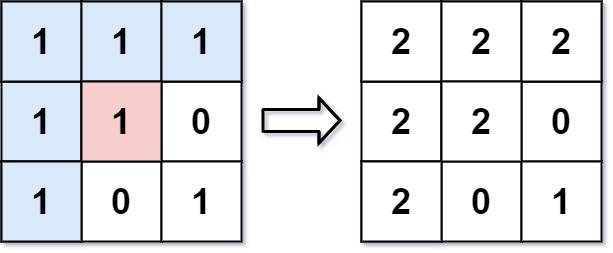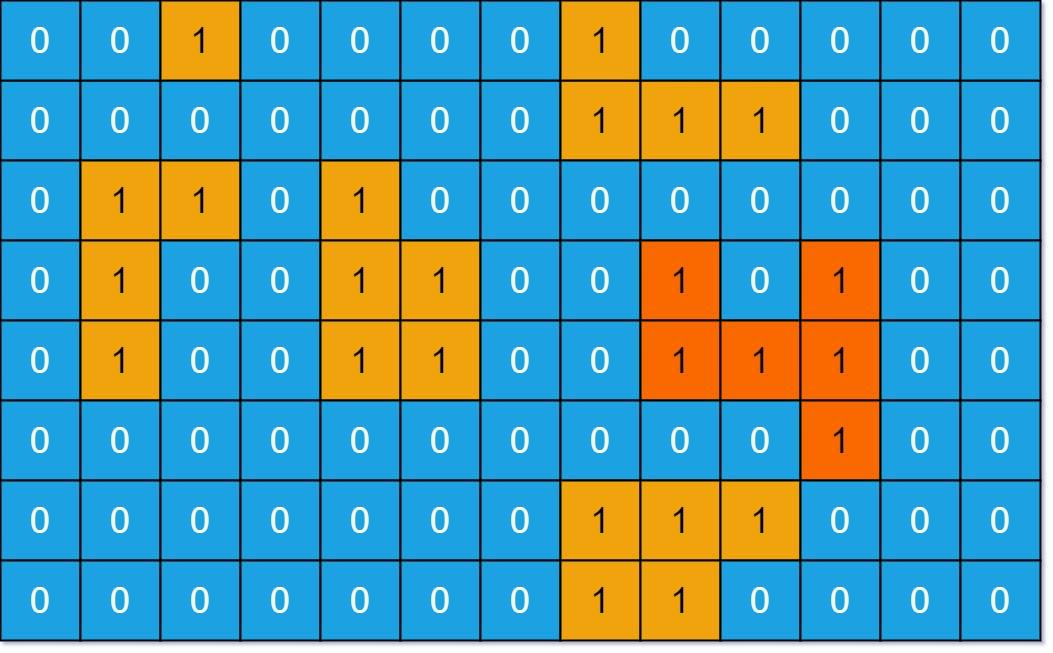# 2022-05-21

## ​733. Flood Fill​

### Description

An image is represented by an `m x n` integer grid `image` where `image[i][j]` represents the pixel value of the image.
You are also given three integers `sr`, `sc`, and `newColor`. You should perform a flood fill on the image starting from the pixel `image[sr][sc]`.
To perform a flood fill, consider the starting pixel, plus any pixels connected 4-directionally to the starting pixel of the same color as the starting pixel, plus any pixels connected 4-directionally to those pixels (also with the same color), and so on. Replace the color of all of the aforementioned pixels with `newColor`.
Return the modified image after performing the flood fill.
Example 1:Input: image = [[1,1,1],[1,1,0],[1,0,1]], sr = 1, sc = 1, newColor = 2
Output: [[2,2,2],[2,2,0],[2,0,1]]
Explanation: From the center of the image with position (sr, sc) = (1, 1) (i.e., the red pixel), all pixels connected by a path of the same color as the starting pixel (i.e., the blue pixels) are colored with the new color.
Note the bottom corner is not colored 2, because it is not 4-directionally connected to the starting pixel.
Example 2:
Input: image = [[0,0,0],[0,0,0]], sr = 0, sc = 0, newColor = 2
Output: [[2,2,2],[2,2,2]]
Constraints:
• `m == image.length`
• `n == image[i].length`
• `1 <= m, n <= 50`
• `0 <= image[i][j], newColor < 2^16`
• `0 <= sr < m`
• `0 <= sc < n`

### Solution

#### Approach #0: DFS

var (
dx = []int{0, 1, 0, -1}
dy = []int{1, 0, -1, 0}
)
func floodFill(image [][]int, sr int, sc int, newColor int) [][]int {
if image[sr][sc] != newColor {
originColor := image[sr][sc]
dfs(image, sr, sc, originColor, newColor)
}
return image
}
func dfs(image [][]int, sr int, sc int, originColor, newColor int) {
if image[sr][sc] == originColor {
image[sr][sc] = newColor
for i := 0; i < 4; i++ {
newSr, newSc := sr+dx[i], sc+dy[i]
if newSr >= 0 && newSr < len(image) && newSc >= 0 && newSc < len(image) {
dfs(image, newSr, newSc, originColor, newColor)
}
}
}
}

#### Approach #1: BFS

var (
dx = []int{0, 1, 0, -1}
dy = []int{1, 0, -1, 0}
)
func floodFill(image [][]int, sr int, sc int, newColor int) [][]int {
if image[sr][sc] == newColor {
return image
}
originColor := image[sr][sc]
image[sr][sc] = newColor
queue := [][]int{[]int{sr, sc}}
for i := 0; i < len(queue); i++ {
for j := 0; j < 4; j++ {
x, y := queue[i]+dx[j], queue[i]+dy[j]
if x >= 0 && x < len(image) && y >= 0 && y < len(image) && image[x][y] == originColor {
image[x][y] = newColor
queue = append(queue, []int{x, y})
}
}
}
return image
}

## ​695. Max Area of Island​

### Description

You are given an `m x n` binary matrix `grid`. An island is a group of `1`'s (representing land) connected 4-directionally (horizontal or vertical.) You may assume all four edges of the grid are surrounded by water.
The area of an island is the number of cells with a value `1` in the island.
Return the maximum area of an island in `grid`. If there is no island, return `0`.
Example 1:Input: grid = [[0,0,1,0,0,0,0,1,0,0,0,0,0],[0,0,0,0,0,0,0,1,1,1,0,0,0],[0,1,1,0,1,0,0,0,0,0,0,0,0],[0,1,0,0,1,1,0,0,1,0,1,0,0],[0,1,0,0,1,1,0,0,1,1,1,0,0],[0,0,0,0,0,0,0,0,0,0,1,0,0],[0,0,0,0,0,0,0,1,1,1,0,0,0],[0,0,0,0,0,0,0,1,1,0,0,0,0]]
Output: 6
Explanation: The answer is not 11, because the island must be connected 4-directionally.
Example 2:
Input: grid = [[0,0,0,0,0,0,0,0]]
Output: 0
Constraints:
• `m == grid.length`
• `n == grid[i].length`
• `1 <= m, n <= 50`
• `grid[i][j]` is either `0` or `1`.

### Solution

#### Approach #0: BFS

var (
dx = []int{0, 1, 0, -1}
dy = []int{1, 0, -1, 0}
)
func maxAreaOfIsland(grid [][]int) int {
if len(grid) == 0 {
return 0
}
m, n := len(grid), len(grid)
res := 0
for i := 0; i < m; i++ {
for j := 0; j < n; j++ {
if grid[i][j] == 0 {
continue
}
grid[i][j] = 0
queue := [][]int{{i, j}}
area := 1
for ii := 0; ii < len(queue); ii++ {
cell := queue[ii]
for jj := 0; jj < 4; jj++ {
x, y := cell+dx[jj], cell+dy[jj]
if x >= 0 && x < m && y >= 0 && y < n && grid[x][y] > 0 {
grid[x][y] = 0
area++
queue = append(queue, []int{x, y})
}
}
}
res = max(res, area)
}
}
return res
}
func max(a, b int) int {
if a > b {
return a
}
return b
}

#### Approach #1: DFS (Recursive)

var (
dx = []int{0, 1, 0, -1}
dy = []int{1, 0, -1, 0}
)
func maxAreaOfIsland(grid [][]int) int {
if len(grid) == 0 {
return 0
}
res := 0
for i := 0; i < len(grid); i++ {
for j := 0; j < len(grid); j++ {
if grid[i][j] == 0 {
continue
}
area := dfs(grid, i, j)
res = max(res, area)
}
}
return res
}
func dfs(grid [][]int, x, y int) int {
grid[x][y] = 0
res := 1
for i := 0; i < 4; i++ {
xx, yy := x+dx[i], y+dy[i]
if xx >= 0 && xx < len(grid) && yy >= 0 && yy < len(grid) && grid[xx][yy] > 0 {
res = res + dfs(grid, xx, yy)
}
}
return res
}
func max(a, b int) int {
if a > b {
return a
}
return b
}

#### Approach #2: DFS + Stack (Iterative)

var (
dx = []int{0, 1, 0, -1}
dy = []int{1, 0, -1, 0}
)
func maxAreaOfIsland(grid [][]int) int {
if len(grid) == 0 {
return 0
}
res := 0
for i := 0; i < len(grid); i++ {
for j := 0; j < len(grid); j++ {
s := [][]int{{i, j}}
area := 0
for len(s) > 0 {
cell := s[len(s)-1]
s = s[:len(s)-1]
x, y := cell, cell
if x < 0 || x >= len(grid) || y < 0 || y >= len(grid) || grid[x][y] == 0 {
continue
}
grid[x][y] = 0
area++
for i := 0; i < 4; i++ {
xx, yy := x+dx[i], y+dy[i]
s = append(s, []int{xx, yy})
}
}
res = max(res, area)
}
}
return res
}
func max(a, b int) int {
if a > b {
return a
}
return b
}